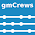### What's Wrong with Risk Matrices?

Risk is equal to probability times consequence. The Wikipedia entry for risk matrix has a reference to an article by Tony Cox titled What's Wrong with Risk Matrices? Unfortunately, the article is behind a paywall. So as far as I am concerned, it doesn't exist. But I was able to google a blog posting about the article.

Interesting was how Cox constructed a risk matrix and then drew lines of constant risk on it. Something like this:
The lines are hyperbolas with the axes as asymptotes.

According to the blog posting referenced above: "Cox shows that the qualitative risk ranking provided by a risk matrix will agree with the quantitative risk ranking only if the matrix is constructed according to certain general principles."

Before examining these general principles, and let me be clear that I fundamentally disagree with these principles, first things first.

I have always viewed risk matrices as having log-log scales. (And I'm not the only one looking at risk matrices this way.) Something like this:

Notice the constant values of risk are all straight lines with a slope of minus one. And note the risk contours in this example are separated by an order of magnitude, not just a doubling of risk value as in the previous figure. This means it is easier to represent a wider range of probabilities and consequence scenarios (measurable in dollar amounts) using a log-log scale.

But the most important reason why I think it is better to use a log-log scale is because risk categorization is subjective. And I believe that where possible subjective judgments, like risk category, should be measured in decibels. As I have written about in a previous post: The decibel is a log scale that simplifies overall power gain/loss calculations and is convenient for ratios of numbers that differ by orders of magnitude. Additionally, log scales have been useful in describing the potential of certain physical phenomenon (e.g., earthquakes) and human perceptions (e.g., hearing). Thus, log scales can be useful when performing risk assessments and other related quality assurance activities.

Next time, a rather loud (pun intended) criticism of Cox's general risk matrix principles. :-)

1.AnonymousMay 15, 2013

Cox's results depend only on ordinal properties, so log-transforming the axes does not affect them.

1.I have a couple of issues with your assertion that Cox's result depends only on ordinal properties.

Risk is probability times consequence. And the exact same thing as consequence times probability. However, according to the Wikipedia article on ordinal arithmetic, ordinal multiplication is not communicative. So risk is not an ordinal number.

As I mentioned, I don't have access to the original article since it's behind a pay-wall. So I am relying on Kailash Awati's "Eight to Late" blog post titled Cox’s risk matrix theorem and its implications for project risk management. (To anybody who hasn't read it, I highly recommend reading it. I link to it in my post above.)

It states Cox’s Second Lemma: if a risk matrix satisfies weak consistency and has at least two colours (green in lower left and red in upper right, if axes are oriented to depict increasing probability and impact), then no red cell can occur in the bottom row or left column of the matrix.

The proof of this Lemma (according to Awati) is based on the curved, hyperbolic shape of the iso-risk contours drawn on the risk matrix. However, as you can see from the figures above, these curved hyperbolas become straight, diagonal lines on a log-log scale.

But as I made clear in my follow-up blog posting, this is not my big complaint with Cox's approach.